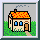## What is Pascal's Triangle?

In the twelfth century both Persian and Chinese mathematicians were working on a so called arithmetic triangle which is relatively easily constructed and which gives the coefficients of the expansion of the algebraic expression (a + b)n for different integer values of n. [3, pp 204 and 242] Here's how it works: